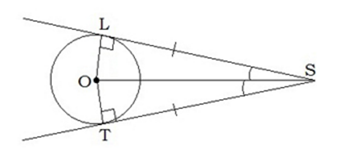##### KAPEC GEOTASK?
• The learning process is entertaining and exciting
• The solved tasks turn into coordinates
• The chance to follow your progress
• Along with your schoolmates and friends, go on an adventure, searching for hideouts
• E-mail:

# 3067.1. Tangent properties and signs.

#### Write the characteristics of a tangent, if it shows, that they are equal! (the angle sign is optional, from the center point in a clockwise)=

#### Next task: 3063. Tangent properties and signs.

Grade:
8
Subject
Geometry
Theme
Circle, center angle and indrawn angle.
Subtheme
Tangent properties and signs.

#### Solve tasks to find hidden Geo point coordinates

1. Physics: Amount of heat.
2. Chemistry: Atoms un ķīmiskie elementi.
3. Physics: Substances structure.
4. Algebra: Albraic fraction.
5. Algebra: Median
6. Chemistry: Substances multiplicity.
7. Algebra: Reverse proportionality concept.
8. Algebra: Actual numbers.
9. Physics: Amount of heat.
10. Algebra: Square root extraction